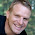### CFD: FEM vs FDM vs FVM

Comparison of the three methods is difficult, primarily due to the many variations of all three methods. FVM and FDM provide discrete solutions, while FEM provides a continuous (up to a point) solution.

Finite Difference Method – FDM
• FDM discretization is based upon the differential form of the PDE to be solved.
• Each derivative is replaced with an approximate difference formula (that can generally be derived from a Taylor series expansion).
• The computational domain is usually divided into hexahedral cells (the grid), and the solution will be obtained at each nodal point.
• The FDM is easiest to understand when the physical grid is Cartesian, but through the use of curvilinear transforms the method can be extended to domains that are not easily represented by brick-shaped elements.
• The discretization results in a system of equation of the variable at nodal points, and once a solution is found, then we have a discrete representation of the solution.
• Mostly for defined geometries which could be represented by structured grids
• One of the deviations in the case of FDM would come from approximating the higher order terms in Taylor’s series which is used to linearize the PDE. This is called as truncation error.
• Other important differentiating factor of FDM compared to FEM and FVM is that it is based on the differential form of governing equations. FEM and FVM are based on the integral form or weak formulation.
Finite Element Method – FEM
• FEM discretization is based upon a piecewise representation of the solution in terms of specified basis functions.
• The computational domain is divided up into smaller domains (finite elements) and the solution in each element is constructed from the basis functions.
• The actual equations that are solved are typically obtained by restating the conservation equation in weak form: the field variables are written in terms of the basis functions, the equation is multiplied by appropriate test functions, and then integrated over an element.
• Discretizes the domain into finite elements and calculates the properties in every node.
• Shape functions or basis functions are used to interpolate inside the finite element, the solutions obtained at these nodes. As a result, the partial differential equations are converted into a set of algebraic equations which are comparatively easier to solve.
• FEM is the most commonly used and it is efficient for all geometries including ones with complicated shapes and features
Finite Volume Method – FVM
• FVM discretization is based upon an integral form of the PDE to be solved (i.e governing eq.).
• The PDE is written in a form which can be solved for a given finite volume (or cell).
• The computational domain is discretized into finite volumes and then for every volume the governing equations are solved.
• The resulting system of equations usually involves fluxes of the conserved variable, and thus the calculation of fluxes is very important in FVM. The basic advantage of this method over FDM is it does not require the use of structured grids, and the effort to convert the given mesh in to structured numerical grid internally is completely avoided. The properties are calculated for every cell instead of a node
• Based on the integral form of conservation laws, it can handle discontinuities in solutions. In simple terms, what comes in, must go out.
• FVM approximates the value of the integral on the reference cell

1.2.